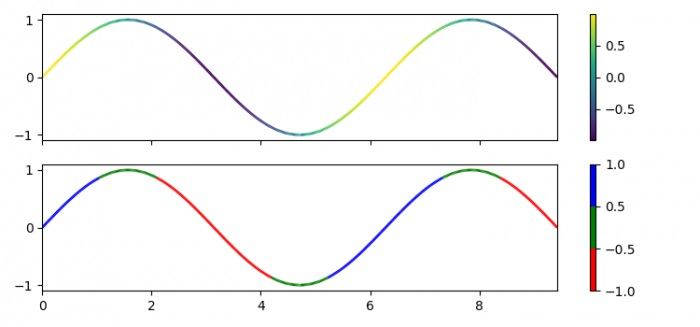# How to vary the line color with data index for line graph in matplotlib?

To have the line color vary with the data index for a line graph in matplotlib, we can take the following steps −

## Steps

• Set the figure size and adjust the padding between and around the subplots.

• Create x and y data points using numpy.

• Get smaller limit, dydx.

• Get the points and segments data points using numpy.

• Create a figure and a set of subplots.

• Create a class which, when called, linearly normalizes data into some range.

• Set the image array from numpy array *A*.

• Set the linewidth(s) for the collection.

• Set the colorbar for axis 1.

• Generate Colormap object from a list of colors i.e r, g and b.

• Repeat steps 6, 7, 8, 9 and 10.

• Set the limit of the X and Y axes.

• To display the figure, use show() method.

## Example

import numpy as np
import matplotlib.pyplot as plt
from matplotlib.collections import LineCollection
from matplotlib.colors import ListedColormap, BoundaryNorm

plt.rcParams["figure.figsize"] = [7.50, 3.50]
plt.rcParams["figure.autolayout"] = True

x = np.linspace(0, 3 * np.pi, 500)
y = np.sin(x)

dydx = np.cos(0.5 * (x[:-1] + x[1:]))
points = np.array([x, y]).T.reshape(-1, 1, 2)
segments = np.concatenate([points[:-1], points[1:]], axis=1)

fig, axs = plt.subplots(2, 1, sharex=True, sharey=True)
norm = plt.Normalize(dydx.min(), dydx.max())

lc = LineCollection(segments, cmap='viridis', norm=norm)
lc.set_array(dydx)
lc.set_linewidth(2)

fig.colorbar(line, ax=axs)
cmap = ListedColormap(['r', 'g', 'b'])
norm = BoundaryNorm([-1, -0.5, 0.5, 1], cmap.N)

lc = LineCollection(segments, cmap=cmap, norm=norm)
lc.set_array(dydx)
lc.set_linewidth(2)

fig.colorbar(line, ax=axs)

axs.set_xlim(x.min(), x.max())
axs.set_ylim(-1.1, 1.1)

plt.show()

## Output

It will produce the following output −